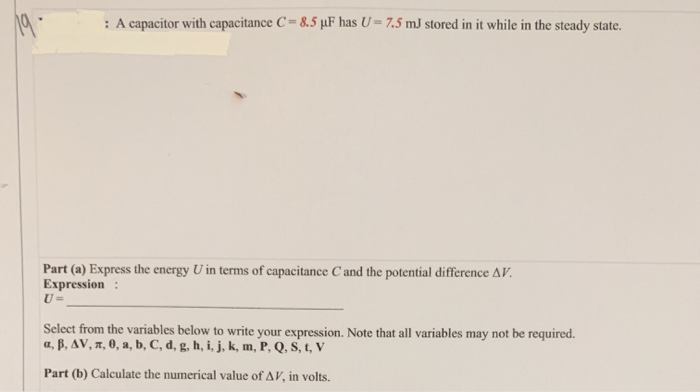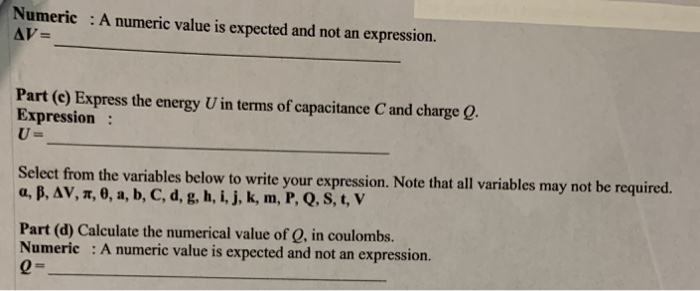# Question Solved1 Answer: A capacitor with capacitance C 8.5 uF has U 7.5 mJ stored in it while in the steady state. Part (a) Express the energy U in terms of capacitance C and the potential difference AV Expression U= Select from the variables below to write your expression. Note that all variables may not be required. a, B, AV, T, 0, a, b, C, d, g, h, i, j, k, m, P, Q, S, t, V : A capacitor with capacitance C 8.5 uF has U 7.5 mJ stored in it while in the steady state. Part (a) Express the energy U in terms of capacitance C and the potential difference AV Expression U= Select from the variables below to write your expression. Note that all variables may not be required. a, B, AV, T, 0, a, b, C, d, g, h, i, j, k, m, P, Q, S, t, V Part (b) Calculate the numerical value of AV, in volts Numeric A numeric value is expected and not an expression. AV= Part (c) Express the energy U in terms of capacitance C and charge Q. Expression: U = Select from the variables below to write your expression. Note that all variables may not be required. a, B, AV, , 0, a, b, C, d, g, h, i, j, k, m, P, Q, S, t, V Part (d) Calculate the numerical value of Q, in coulombs. Numeric A numeric value is expected and not an Q= expression.Transcribed Image Text: : A capacitor with capacitance C 8.5 uF has U 7.5 mJ stored in it while in the steady state. Part (a) Express the energy U in terms of capacitance C and the potential difference AV Expression U= Select from the variables below to write your expression. Note that all variables may not be required. a, B, AV, T, 0, a, b, C, d, g, h, i, j, k, m, P, Q, S, t, V Part (b) Calculate the numerical value of AV, in volts Numeric A numeric value is expected and not an expression. AV= Part (c) Express the energy U in terms of capacitance C and charge Q. Expression: U = Select from the variables below to write your expression. Note that all variables may not be required. a, B, AV, , 0, a, b, C, d, g, h, i, j, k, m, P, Q, S, t, V Part (d) Calculate the numerical value of Q, in coulombs. Numeric A numeric value is expected and not an Q= expression.
More
Transcribed Image Text: : A capacitor with capacitance C 8.5 uF has U 7.5 mJ stored in it while in the steady state. Part (a) Express the energy U in terms of capacitance C and the potential difference AV Expression U= Select from the variables below to write your expression. Note that all variables may not be required. a, B, AV, T, 0, a, b, C, d, g, h, i, j, k, m, P, Q, S, t, V Part (b) Calculate the numerical value of AV, in volts Numeric A numeric value is expected and not an expression. AV= Part (c) Express the energy U in terms of capacitance C and charge Q. Expression: U = Select from the variables below to write your expression. Note that all variables may not be required. a, B, AV, , 0, a, b, C, d, g, h, i, j, k, m, P, Q, S, t, V Part (d) Calculate the numerical value of Q, in coulombs. Numeric A numeric value is expected and not an Q= expression.